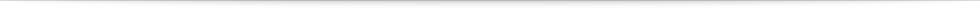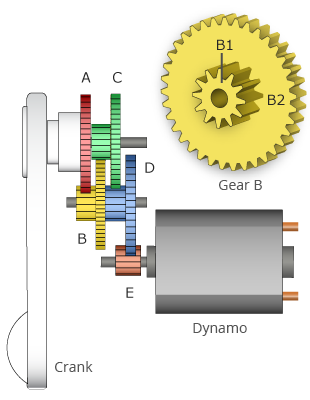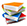Teach
With
Bigshot
 Prev Introduction      Gears      Gearbox      Electromagnetism Dynamo      Battery      Circuit      Illustrations Next### GearboxFigure 3: Bigshot's gearbox

To generate enough power in a few seconds to take a photo, Bigshot's dynamo must be spun at thousands of rotations per minute. However, it is humanly impossible to rotate the crank at such a speed.

One solution is to interconnect the crank and dynamo using two gears. However, in order to boost the rotation speed by a factor of say 100, the first gear has to be 100 times as big as the second one. That would make the camera huge!

This problem can be solved by using multiple gears. A series of intermeshed gears of different sizes is called a gearbox. Bigshot's gearbox, shown in Figure 3, uses eight gears that work together to convert the slow rotation of the hand crank to a very fast rotation of the dynamo shaft, without requiring the camera to be unreasonably large. The inset at the top-right corner of Figure 3 shows a compound gear B. It consists of two separate gears (B1 and B2) that are not meshed but rather fixed together. Rotating either one of the gears rotates the other at the same speed. Gears C and D are also compound gears, and are composed of gears C1 and C2, and D1 and D2, respectively. Gear A is two times larger than gear B1. The remaining larger gears (B2, C2 and D2) are three times as large as the smaller gears (C1, D1 and E).

Gear A is directly attached to the crank and hence rotates at the same speed as the crank. It is meshed with B1, which rotates twice as fast as A. Since B2 is fixed to B1, it also rotates at this speed. The speed further increases three times when B2 drives C1. Now we can see that each time the rotation transfers from one compound gear to the next, the speed increases by a factor of three. This means that by the time the rotation of the crank transfers through the four gears to reach the dynamo shaft, the speed would have increased by a factor of 2×3×3×3=54 times. That is, a single rotation of the crank makes the dynamo spin 54 times!

For example, if we rotate the crank at 30 rotations per minute, the dynamo spins at 30×54 = 1620 rotations per minute, which is a high enough speed to generate the power needed for Bigshot to take a photo.

 Prev Next###References

  D. W. Dudley, Handbook of Practical Gear Design. CRC, 1994.  B. Marshall, "How Gears Ratios Work." HowStuffWorks.com. Nov 20, 2000. Available: http://www.howstuffworks.com/gears.htm